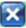# Category:Weighting

(Redirected from Weight)
Related Online Training modules
Applying Weights
Setting a Weight in the Data Tab
Setting a Weight in the Variables and Questions Tab
Creating a Weight with 1 Variable
Creating a Weight with 2 Variables
Creating a Weight by Wave
Generally it is best to access online training from within Q by selecting Help : Online Training

Weighting, also known as sampling balancing, is used to make adjust the results of studies to make them more representative (e.g., if a study has 20% men, but the population has 50%, weighting can be used to bring the results of the study into line with the population).

Weights are applied in the Weight drop-down at the bottom of the screen. Different weights can be applied to different tables in a project. Pressto remove a weight.

## Interpreting the information about weighting at the bottom of the screen

In addition to the drop-down, the presence of weighting is also reflected in the sample size description at the bottom of the screen.

base n = from 151 to 215; total n = 223; 72 missing; effective sample size = 156 (70%); 80% filtered out


This description indicates that :

• The sample size varies for different cells in the table, the cell with the smallest sample size being 151 while the largest cell has a sample size of 215. (We can see each cell’s sample size by selecting Base n from Statistics – Cells (see Statistics).
• The total sample size of the table is 223 (i.e., if there were no missing data, each cell would be based on a sample size of 223).
• Each cell has up to 72 missing values (i.e., 223 minus 151).
• The Effective Sample Size is 156 (this is computed on the largest base n of 215). In most instances, weighting causes a decrease in the statistical significance of results. The effective sample size is a measure of the precision of the survey (e.g., even if you have a sample of 1,000 people, an effective sample size of 100 would indicate that the weighted sample is no more robust than a well-executed, un-weighted, simple random sample of 100 people).
• The weighted sample size of 223 has the same precision as an un-weighted sample of 70% the size (i.e., 156 / 223) for this table.
• The percentage shown in brackets indicates the ratio of the effective sample size to the total sample size. A score of 100% indicates that the weighting has caused no loss of precision.
• The final statistic (80% filtered out) shows the proportion of the total number of observations in the study that is not available for the analysis due to a filter having been applied to the table.

## Weights, sample size and population

In commercial market research, weights generally have two roles: to correct for bias in the sampling (e.g., over-representation of a particular segment) and to gross up the sample size to match known population totals (e.g., the number of people in a country). Q accommodates both of these roles. When a weight is applied, all percentages and averages automatically take the weight into account. Estimates of the population size can be obtained by selecting Population from Statistics – Cells, Column Population in Statistics – Below and Row Population in Statistics – Right.